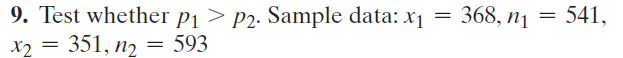×
Get Full Access to Statistics: Informed Decisions Using Data - 5 Edition - Chapter 11.1 - Problem 9
Get Full Access to Statistics: Informed Decisions Using Data - 5 Edition - Chapter 11.1 - Problem 9

×

# ?In Problems 9–12, conduct each test at the = 0.05 level of significance by determining (a) the null and alternative hypotheses, (b) the test statistiISBN: 9780134133539 240

## Solution for problem 9 Chapter 11.1

Statistics: Informed Decisions Using Data | 5th Edition

• Textbook Solutions
• 2901 Step-by-step solutions solved by professors and subject experts
• Get 24/7 help from StudySoup virtual teaching assistantsStatistics: Informed Decisions Using Data | 5th Edition

4 5 1 328 Reviews
29
0
Problem 9

In Problems 9–12, conduct each test at the= 0.05 level of significance by determining (a) the null and alternative hypotheses, (b) the test statistic, (c) the critical value, and (d) the P-value. Assume that the samples were obtained independently using simple random sampling.

9. Test whether p1 7 p2. Sample data: x1 = 368, n1 = 541, x2 = 351, n2 = 593Step-by-Step Solution:

Step 1 of 5) In Problems 9–12, conduct each test at the = 0.05 level of significance by determining (a) the null and alternative hypotheses, (b) the test statistic, (c) the critical value, and (d) the P-value. Assume that the samples were obtained independently using simple random sampling. 9. Test whether p1 7 p2. Sample data: x1 = 368, n1 = 541, x2 = 351, n2 = 593. It is important to understand that subjective probabilities are perfectly legitimate and are often the only method of assigning likelihood to an outcome. As another example, a financial reporter may ask an economist about the likelihood the economy will fall into recession next year. Again, we cannot conduct an experiment n times to obtain a relative frequency. The economist must use her knowledge of the current conditions of the economy and make an educated guess about the likelihood of a recession.

Step 2 of 2

##### ISBN: 9780134133539

This full solution covers the following key subjects: . This expansive textbook survival guide covers 88 chapters, and 2422 solutions. This textbook survival guide was created for the textbook: Statistics: Informed Decisions Using Data, edition: 5. The full step-by-step solution to problem: 9 from chapter: 11.1 was answered by , our top Statistics solution expert on 01/15/18, 03:19PM. Statistics: Informed Decisions Using Data was written by and is associated to the ISBN: 9780134133539. Since the solution to 9 from 11.1 chapter was answered, more than 219 students have viewed the full step-by-step answer. The answer to “?In 9–12, conduct each test at the = 0.05 level of significance by determining (a) the null and alternative hypotheses, (b) the test statistic, (c) the critical value, and (d) the P-value. Assume that the samples were obtained independently using simple random sampling.9. Test whether p1 7 p2. Sample data: x1 = 368, n1 = 541, x2 = 351, n2 = 593” is broken down into a number of easy to follow steps, and 62 words.

## Discover and learn what students are asking

Calculus: Early Transcendental Functions : Area and Arc Length in Polar Coordinates
?In Exercises 5-16, find the area of the region. One petal of $$r=4 \sin 3 \theta$$

Statistics: Informed Decisions Using Data : Introduction to the Practice of Statistics
?A(n)_________ is a person or object that is a member of the population being studied.

Statistics: Informed Decisions Using Data : Properties of the Normal Distribution
?In Problems 25–28, the graph of a normal curve is given. Use the graph to identify the values of ? and ?.

Statistics: Informed Decisions Using Data : Testing the Significance of the Least-Squares Regression Model
?Why don’t we conduct inference on the linear correlation coefficient?

Unlock Textbook Solution